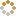﻿ Ningbo University of Technology Online Judge ::  Repeating Characters
• ###  Repeating Characters

• 时间限制: 1000 ms　内存限制: 65535 K
• 问题描述
• For this problem, you will write a program that takes a string of characters, S, and creates a new string of characters, T, with each character repeated R times. That is, R copies of the first character of S, followed by R copies of the second character of S, and so on. Valid characters for S are the QR Code "alphanumeric" characters:
0123456789ABCDEFGHIJKLMNOPQRSTUVWXYZ\$%*+-./:
• 输入
• The first line of input contains a single integer P, (1 <= P <= 1000), which is the number of data sets that follow. Each data set is a single line of input consisting of the data set number N, followed by a space, followed by the repeat count R, (1 <= R <= 8), followed by a space, followed by the string S. The length of string S will always be at least one and no more than 20 characters. All the characters will be from the set of characters shown above.
• 输出
• For each data set there is one line of output. It contains the data set number, N, followed by a single space which is then followed by the new string T, which is made of each character in S repeated R times.
• 样例输入
• ```2
1 3 ABC
2 5 /HTP
```
• 样例输出
• ```1 AAABBBCCC
2 /////HHHHHTTTTTPPPPP
```
• 提示
• `无`
• 来源
• `Greater New York Region`
• 操作
﻿